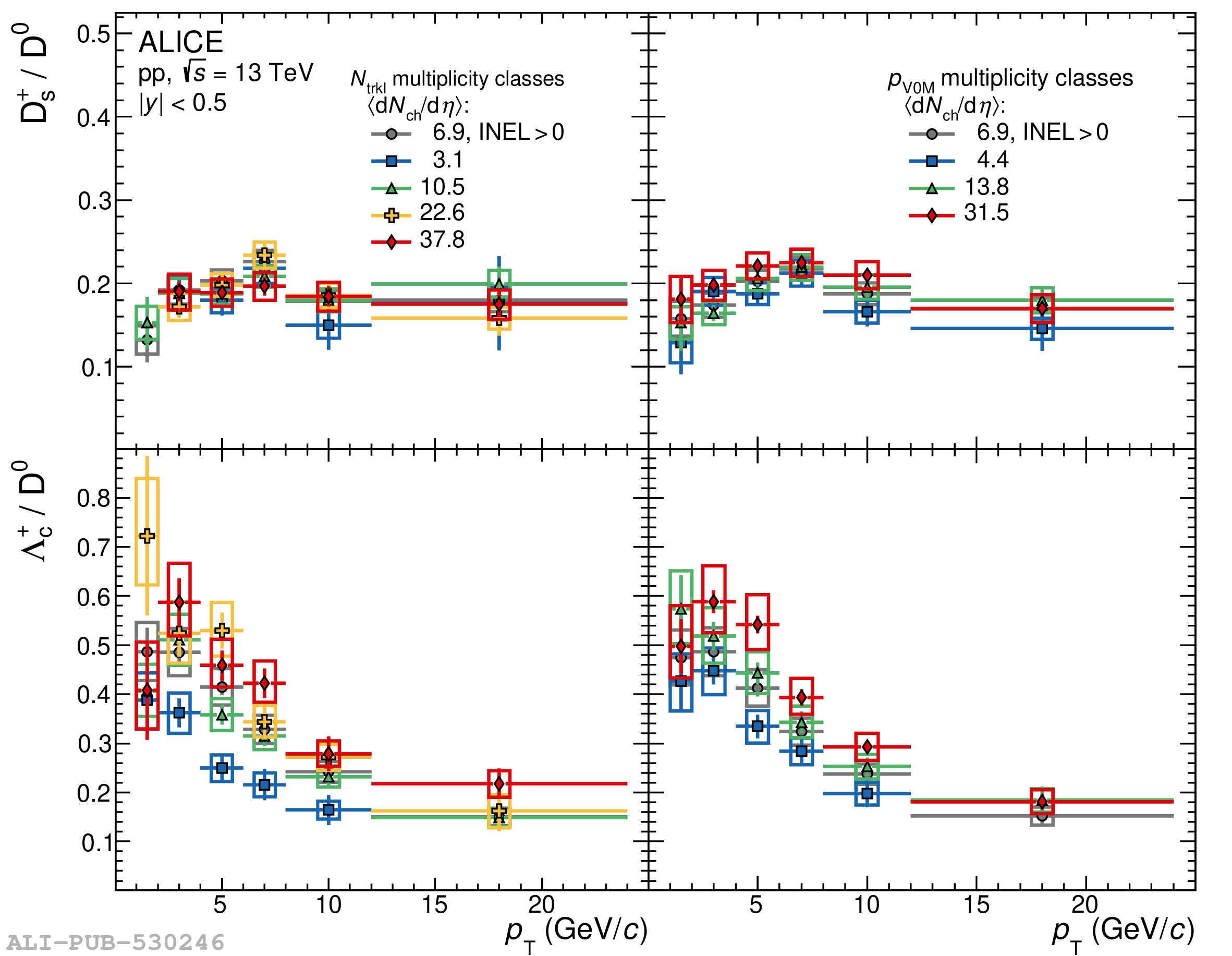# Figure 2

 The ${\rm D_s^+}/{\rm D^0}$ (top) and $\Lambda_{\rm c}^+/{\rm D^0}$ (bottom) ratios measured in pp collisions at $\sqrt{s}=13$ TeV for different multiplicity classes at mid- (left) and forward (right) rapidity.